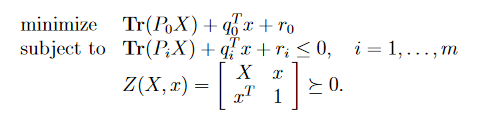# Semidefinite relaxation of non-convex QCQP

Hello,

I am trying to apply the SDR of a non-convex QCQP on my problem. However, the output status is always unbounded, and the constraints are not being satisfied at all. Can someone please explain why does this happen even though the problem is convex with convex constraints?
Thanks.Code:

clear all; clc
%% Physical layer / Hardware parameters
L = 4; %No of Learners
O = 2;
x = 1.2e9; %fk = 1.2Ghz
y = 0.7e9; %fk = 700Mhz
z = 0.5e9; %fk = 500Mhz
fk = zeros(L,1);
fk(1:3:end) = x;
fk(2:3:end) = y;
fk(3:3:end) = z;
fk_lo = repmat(fk,1,O);
W = 5e6; %Node Bandwidth = 100Mhz
alpha = 2; % path loss
sigma2 = 1e-10; % noise variance
g2 = 0.1; %random(‘exp’,0.1); channel gain
Pt = 0.2; % transmission power Watt
mu = 1e-19; % capacity factor for computing energy consumption
zeta = 2; % frequency exponent for computing energy consumption
%% Learning parameters
D = 6e4; % Data size
Gamma_mo = 32;
Smo = 8974080;
F_o = 784;
Gamma_do = 32;
Cmo = 67424160/48;
phi = 0.005; % control parameters == rho/(omega.epsilon2)
eta = 0.01; % learning rate
beta = 0.01; % Lipschitz gradient smootheness
delta = 0.01; % gradient divergence
%% Environment parameters
maxDistance = 50;
d_lo = floor(rand(L,O)*50);
d_lo(d_lo<5) = 5;

A0_lo = 2Gamma_moSmo./(Wlog2(1+ g2d_lo.^(-alpha)Pt/sigma2));
A1_lo = D
F_oGamma_do./(Wlog2(1+ g2*d_lo.^(-alpha)Pt/sigma2)); % dk
A2_lo = D
Cmo./fk_lo; % t
dk

B0_lo = PtA0_lo;
B1_lo = Pt
A1_lo; % dk
B2_lo = Pt
muA2_lo.(fk_lo.^(zeta)); % t*dk
%% Optimization section
c1 = 101.7277;
c2 = -0.9954;
T_max = 600;

G = 1;
tau = 1;

I = eye(L);
learners_orch_code = {};
for i=1:L
c = [];
for j=1:O
c = [ c I(:,i)’];
end

learners_orch_code{i} = c;
end

orch_code = {};
for i=1:O
start = (i-1)*L + 1;
code = [zeros(1,(i-1)*L) ones(1,L) zeros(1,(O-i)*L)];
orch_code{i} = code;
end

Q_0 = zeros(2LO,2LO);
q_0 = [ Greshape(B0_lo,1,LO) zeros(1,LO)];
(Gtaureshape(B2_lo,1,LO) + Greshape(B1_lo,1,LO));
for i=1:L
O
Q_0(L
end

Q_c = zeros(2LO,2LO);
q_c = [Greshape(A0_lo,1,LO) zeros(1,LO)];
(Gtaureshape(A2_lo,1,LO) + Greshape(A1_lo,1,LO));
for i=1:L
O
Q_c(L
end

for i=1:L
code = learners_orch_code{i};
code_matrix = diag(code);
zero = zeros(LO,LO);
Q_i = [zero code_matrix;code_matrix zero];
Q_i = Q_i.Q_c;
q_i = q_c.
[code zeros(1,L*O)];
Q_m{i} = Q_i;
q_m{i} = q_i;
end

for i=1:O
code = orch_code{i};
code_matrix = diag(code);
zero = zeros(LO,LO);
Q_i = [zero code_matrix;code_matrix zero];
Q_i = 0.5*Q_i;
Q_eq{i} = Q_i;
end

n = 2LO;
cvx_begin SDP

variable X(n,n) symmetric;
variable x(n);
minimize(trace(Q_0X) + q_0x)
subject to

for i=1:L
trace(Q_m{i}*X) + q_m{i}x - 600 <= 0;
end
for i=1:O
-[orch_code{i} zeros(1,L
O)]x +1 <=0;
-[zeros(1,L
O) orch_code{i}]*x +1 <=0;
end
[X x;x’ 1] >=0;
x<=1;
x>=0;
cvx_end

You should fix the numerical scaling to not have such large and small magnitude numbers. Even if the original QCQP problem is unbounded, you have relazed it, which means that it is less constrained, so perhaps could be unbounded?